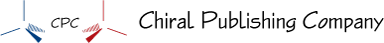﻿ Balancing Equations Help

#Balancing Chemical Equations

 General Steps Consider the first element listed in the first formula in the equation. If this element is mentioned in two or more formulas on the same side of the arrow, skip it until after the other elements are balanced. If this element is mentioned in one formula on each side of the arrow, balance it by placing coefficients in front of one or both of these formulas. Moving from left to right, repeat the process for each element. When you place a number in front of a formula that contains an element you tried to balance previously, recheck that element and put its atoms back in balance. Continue this process until the number of atoms of each element is balanced. The following strategies can be helpful for balancing certain equations. Strategy 1: Often, an element can be balanced by using the subscript for this element on the left side of the arrow as the coefficient in front of the formula containing this element on the right side of the arrow and vice versa (using the subscript of this element on the right side of the arrow as the coefficient in front of the formula containing this element on the left side). Strategy 2: It is sometimes easiest, as a temporary measure, to balance the pure nonmetallic elements (H2, O2, N2, F2, Cl2, Br2, I2, S8, Se8, and P4 ) with a fractional coefficient (1/2, 3/2, 5/2, etc.). If you do use a fraction during the balancing process, you can eliminate it later by multiplying each coefficient in the equation by the fraction’s denominator (usually the number 2). Strategy 3: If polyatomic ions do not change in the reaction, and therefore appear in the same form on both sides of the chemical equation, they can be balanced as if they were single atoms. Strategy 4: If you find an element difficult to balance, leave it for later.
Chiral Publishing Company